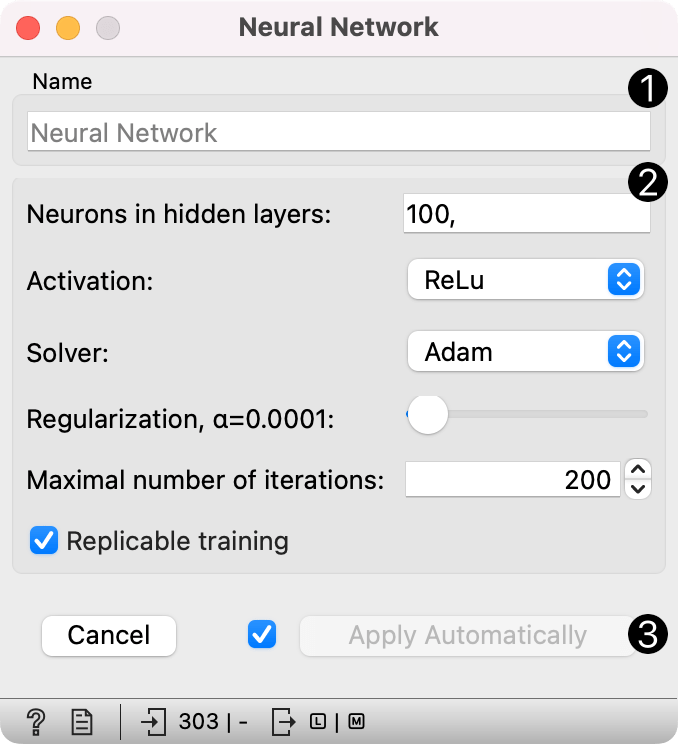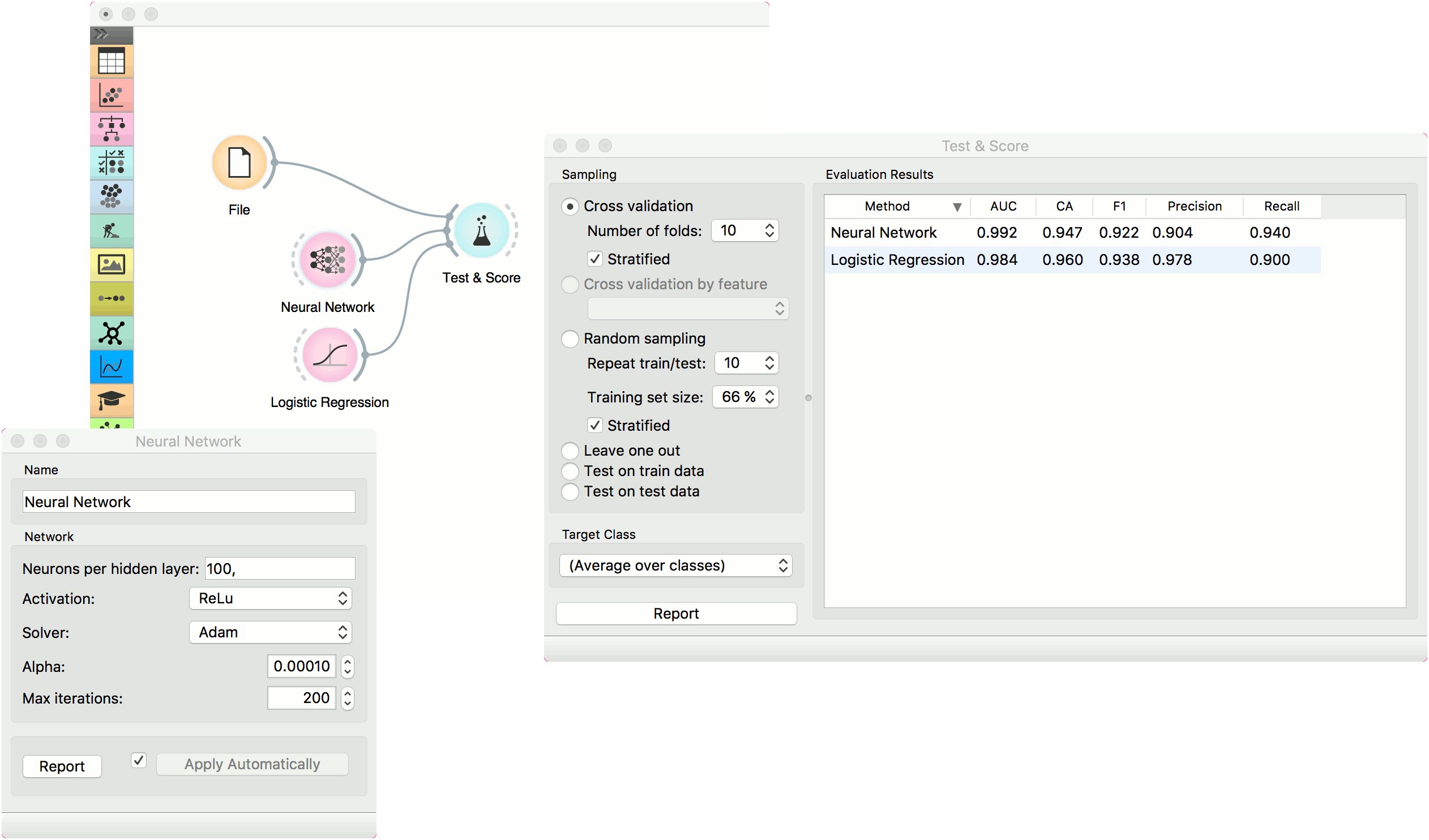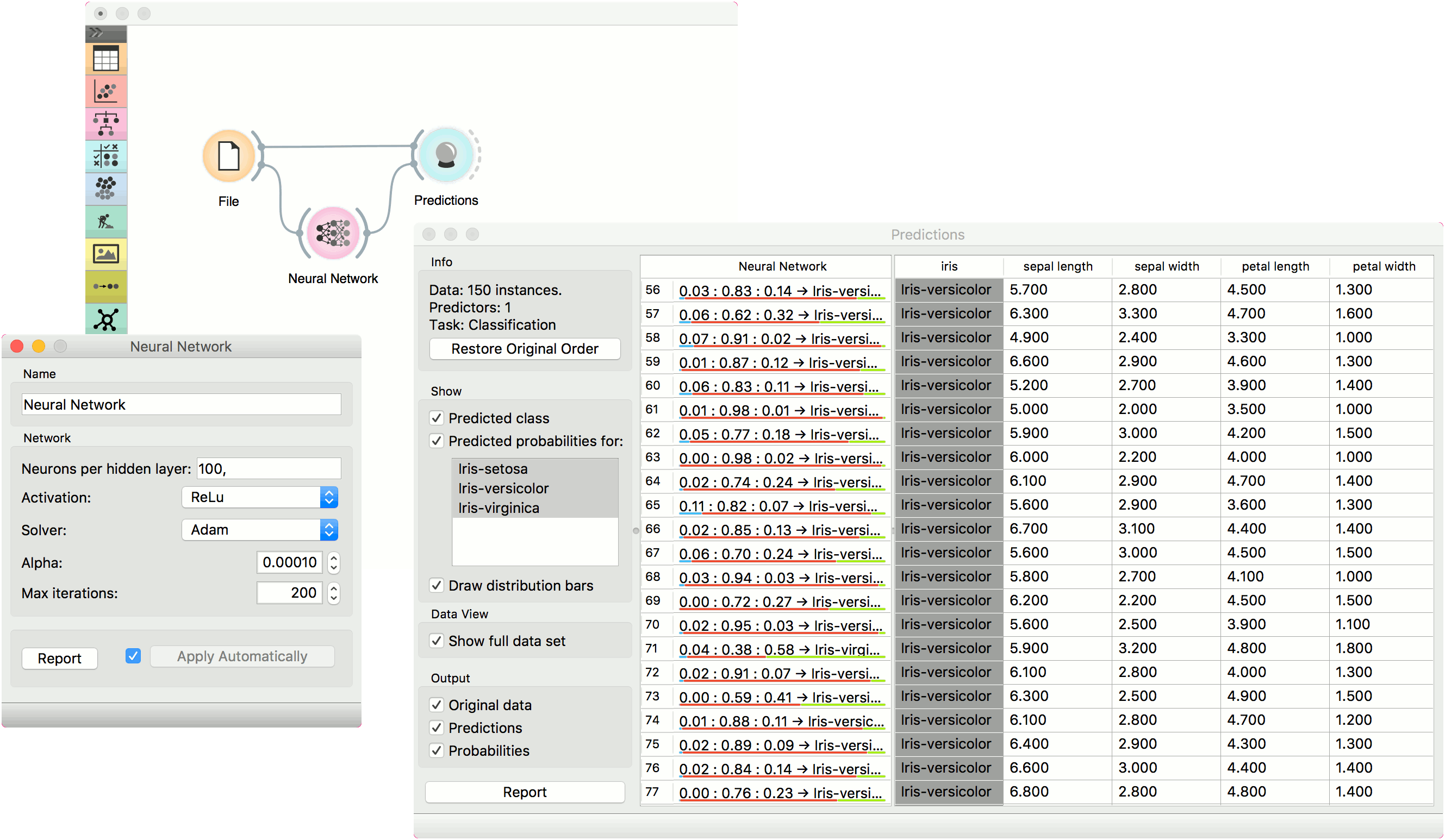# Neural Network

A multi-layer perceptron (MLP) algorithm with backpropagation.

Inputs

• Data: input dataset
• Preprocessor: preprocessing method(s)

Outputs

• Learner: multi-layer perceptron learning algorithm
• Model: trained model

The Neural Network widget uses sklearn's Multi-layer Perceptron algorithm that can learn non-linear models as well as linear.1. A name under which it will appear in other widgets. The default name is "Neural Network".

2. Set model parameters:

• Neurons per hidden layer: defined as the ith element represents the number of neurons in the ith hidden layer. E.g. a neural network with 3 layers can be defined as 2, 3, 2.
• Activation function for the hidden layer:
• Identity: no-op activation, useful to implement linear bottleneck
• Logistic: the logistic sigmoid function
• tanh: the hyperbolic tan function
• ReLu: the rectified linear unit function
• Solver for weight optimization:
• L-BFGS-B: an optimizer in the family of quasi-Newton methods
• Alpha: L2 penalty (regularization term) parameter
• Max iterations: maximum number of iterations

Other parameters are set to sklearn's defaults.

3. Produce a report.

4. When the box is ticked (Apply Automatically), the widget will communicate changes automatically. Alternatively, click Apply.

## Preprocessing

Neural Network uses default preprocessing when no other preprocessors are given. It executes them in the following order:

• removes instances with unknown target values
• continuizes categorical variables (with one-hot-encoding)
• removes empty columns
• imputes missing values with mean values
• normalizes the data by centering to mean and scaling to standard deviation of 1

To remove default preprocessing, connect an empty Preprocess widget to the learner.

## Examples

The first example is a classification task on iris dataset. We compare the results of Neural Network with the Logistic Regression.The second example is a prediction task, still using the iris data. This workflow shows how to use the Learner output. We input the Neural Network prediction model into Predictions and observe the predicted values.